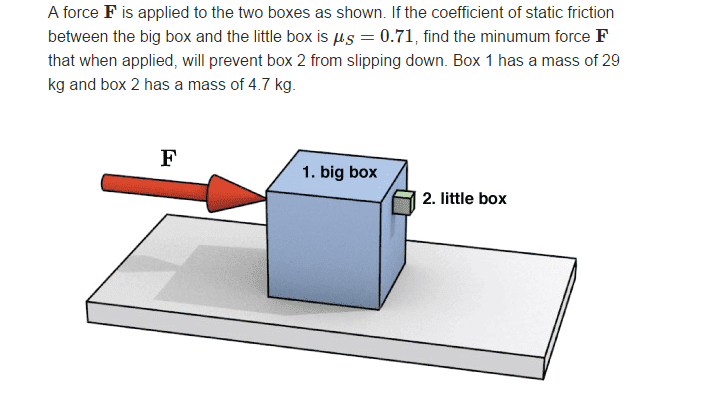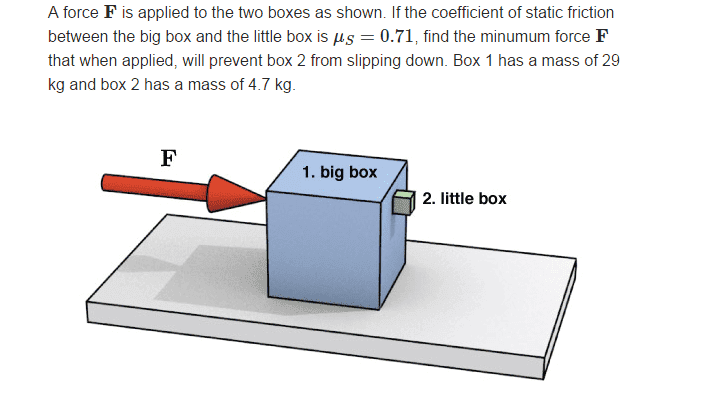# Static Friction of sliding box on plane

## Homework StatementFs = μ FN

## The Attempt at a Solution

Ok, so I drew a free body diagram and tilted it 90 degrees so that Fg of the small box points right and Fstatic points left.

Fg = 4.7 kg x 9.8m/s2 = 46 N

we want Fs to equal 46 N , correct? so we use the formula

Fs = (0.71) x FN

FN being the force of big box on small box

.71 x FN = 46 N

FN = 64.8N

Which is wrong. any help?

ehild
Homework Helper

## Homework StatementFs = μ FN

## The Attempt at a Solution

Ok, so I drew a free body diagram and tilted it 90 degrees so that Fg of the small box points right and Fstatic points left.

Fg = 4.7 kg x 9.8m/s2 = 46 N

we want Fs to equal 46 N , correct? so we use the formula

Fs = (0.71) x FN

FN being the force of big box on small box

.71 x FN = 46 N

FN = 64.8N

Which is wrong. any help?

You calculated the normal force between the boxes, but you need the applied force, F.

billy_joule
What is the required acceleration of box one? How is the mass of box one relevant?

You calculated the normal force between the boxes, but you need the applied force, F.
oh, silly me.

so the acceleration of the small box should be 64.8 / 4.7k which is 13.8 m/s^2

we use F=ma , m being mass of both boxes together and a being 13.8 m/s^2

F = 465 N

ehild
Homework Helper
so the acceleration of the small box should be 64.8 / 4.7k which is 13.8 m/s^2

we use F=ma , m being mass of both boxes together and a being 13.8 m/s^2

F = 465 N
Correct!